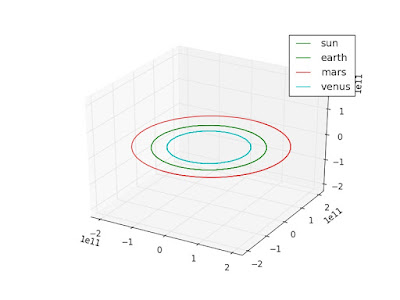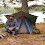## November 16, 2016

### Python N-body Orbit Simulation

Do you ever find yourself thinking "I wish I could do more recreational physics"? If so, today is your lucky day! We're going simulate our very own solar system.

At the end of this tutorial, you should be able to define a hypothetical gravitational system, and graph the paths of the bodies involved over a custom time frame.

This sounds complicated, because usually physics relating to space is considered hard. But it's not that hard! If someone has already broken down the algorithm (which I will do), you just have to understand the rough theory. Then it's no harder than writing a bot. If you've ever studied kinematics, you should also be able to follow along with the physics.

This is also the first in a series of posts. I will be solving this problem again using C++ instead of python, and introducing some High Performance Computing techniques.

If you don't care about theory, you can also skip straight to the program. An intro to python can be found here.

### ELI15 Gravitational Theory

We'll start with the difference between weight and mass. Mass is a measure of the matter that makes up an object, and weight is how much force it feels from gravity. So your weight on the Moon and on Earth are different, but your mass is the same. That's because the Moon has less gravity dragging you down man.

Things with mass naturally pull other things with mass toward them in a straight line. As you get closer, the pulling gets stronger. For example, you feel lighter on the top of Everest than at the base (100 lbs feels like 99.7 lbs).

Now let's talk about orbits. Imagine a bowling ball far above the Earth's surface. The ball is high enough it would take 5 minutes to free fall to the surface. To get into orbit, you just need to ball to move "sideways" fast enough in 5 minutes that it "misses" the Earth.

In the sketch below, you can see some paths that crash into the Earth, and the orbit that ensues when you go fast enough to miss it.For a more in depth explanation, I would recommend wikipedia's page on Orbital Mechanics, or playing Kerbal Space Program

To calculate our orbits, we pick an object (body), and compute its force of gravity coming from all the other bodies in the simulation. From the force, we can find the current acceleration thanks to Newton's second law (F=ma).

The formula for the acceleration from multiple bodies of mass is:

where aix is acceleration in the x direction for body i, G is the gravitational constant, m is the mass of a body, and x,y,z are the coordinates of a body. The body we are calculating for is index i, and we sum contributions from all other indices j.

The y and z directional accelerations are computed just by changing the final term (x_j  - x_i ) to your desired dimension.

As we already know the velocity and position of the object, knowing the acceleration allows us to calculate the bodies location a small time in the future. The acceleration is always changing though, so the smaller the time step, the more accurate our simulation.

Then we repeat this for all the other bodies, and update the positions simultaneously. This is called the Euler Method (err... actually, the Euler-Cromer method based on comments below).

## The Program

If you like to skip ahead, the complete code can be found here.

To run this tutorial, you need Python 3 (or higher), and matplotlib. Matplotlib is how we will visualize the paths of our orbiting bodies. If you have an Anaconda python distribution, it will already be included, or it can be installed by running:

\$> python -m pip install matplotlib

We start by importing the necessary modules, and creating a point class, and a body class. The point class just holds x,y,z information, and the body class holds mass, location, and velocity data. These will be used throughout the program.

### Single Body Calculation

In the theory section, we talked about how this whole thing hinges around calculating the accleration of a single body as a summed acceleration from all other bodies. In python, this can be implemented as follows:

The calculate_single_body_acceleration function takes a list of bodies, and the index of the target_body. Then we iterate through all the external bodies adding up the acceleration of our target body.

### Update velocity and position

With the acceleration known, the next step is to calculate the updated velocity. This is done for all bodies before changing the positions, as we want to keep the time steps synchronized.

The new velocity is calculated simply by multiplying the acceleration with the time step and adding it to the current velocity.

With all velocities up to date, we can now update the location for all bodies by calculating the distance traveled in the time step (velocity x time), and adding it to the current position.

These can be combined into a single callable compute_gravity_step function:

### Simulating orbits

While we're running the simulation, the real valuable information we're finding is the x,y,z locations of our bodies. So to run the simulation, we simply repeat the calculations outlined above for a desired number of steps, and save a history of locations.

This only requires a list of input bodies(with some optional parameters), and returns a list of location histories.

### Visualizing locations

From this history, here is the simplest visualization I could come up with:

This plots a 3d line graph with equal axis scales, sized to the largest dimension. If you provide a filename, it will save to file, otherwise you'll get a graphic popup while running.

### Running a simulationOutput from running the simulation, detailing the motion of The Sun, Venus, Earth, and Mars.

Now all the pieces are in place, and we just have to build up some data and run the functions we wrote in previous sections. You can tweak this however you want, but see below for an example of usage.

Once you get it running, have some fun with the bodies to see what sort of wacky and unstable orbits you can create!

For more fun with orbit simulations, checkout my follow up post introducing the RK4 method.

1.Orbit calculations are inherently unstable, while multi-body interactions are dynamically chaotic. Its a great numerical exercise.

2.Is it possible we are missing the function compute_gravity_step(). The example above doesn't include it it seems. I'm getting an error saying: global name 'compute_gravity_step' is not defined.

3.TD, you're absolutely right. Compute_gravity_step was included in the full code link, but missing from the walkthrough. This has now been added. Thank you for the note.

4.can you do a script that run the simulation of the conjunction orbit of earth-mars with python ?

5.Yes, it is just a matter of using the right initial conditions.

6.The walkthrough says "import matplotlib.pyplot as py" instead of "as plot"

7.I don't know where you're seeing that, for me its:
"import matplotlib.pyplot as plot"

8.In actual fact you have inadvertently implemented the (first-order) Euler-Cromer method, which is a symplectic (but not time-symmetric) first-order integrator. Although less accurate this will have better long-term energy stability than even RK4.

I will try to remember to post a one-line adjustment to implement the second-order Stormer-Verlet (leapfrog) method instead when I get home from work.

9.Thanks Anonymous! I realized my acceleration and locations had staggered states, so is this what makes it Euler-Cromer? I welcome any and all inputs to both the code, and correct language usage.

10.Yes, Euler would require you to store and use the previous velocity/position, so it is actually harder than the symplectic one!

To do a second order symplectic, I think you just need this:

def compute_gravity_step(bodies, time_step = 1):
update_location(bodies, time_step = time_step * 0.5)
compute_velocity(bodies, time_step = time_step)
update_location(bodies, time_step = time_step * 0.5)

You should be able to do an alternative one with the location update as the "filling" too.

11.the code page is not opening could someone send it to me

thanks

12.asem, which code page isn't loading? Everything looks fine to me. Anywhere, here's the github link: https://github.com/benrules2/nbody/tree/master/Python_Orbits

13.This comment has been removed by the author.

14.hello ben

i have a question why when i run the simulation it only shows me only one dot instead of orbits as above

thanks

15.Did anyone have the problem where they ran this simulation and their output was only the lines on a single plane? As far as I can tell I've copied the program over verbatim and I haven't been able to find any mistakes. Instead of the rings, it appears my output is only on a single plane, so ultimately I had figured my issue was with the way the plot was written, however I can't seem to find anything in the matplot literature that would indicate an error.

I apparently dont have access to the full program to differentiate from the version shown here.

I'm still a very novice programmer and I'm trying to learn, so any insight would be hugely helpful.

16.When I click on "the program" I get a Blogger error:

I found the link to the git repo, thanks. And thanks for an interesting post.

17.Thanks Jim! Fixed. Dead links are very frustrating so I appreciate the comment, and I'm glad you enjoyed the post.

18.> Fixed

Great, thank you.

> I'm glad you enjoyed the post.

I did, thank you for putting it up. Although, FWIW, for me, I don't get a picture like the simulation one you have above. I get a picture of the planets and the sun lined up, but no motion.